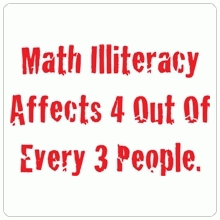Search IntMath
Close

# Math illiteracy

By Murray Bourne, 07 Oct 2006

I came across a CafePress store selling funny math paraphernalia.Text of the image:

"Math illiteracy affects 4 out of every 3 people".

Perhaps this might be a good extra curricular activity, to encourage students to think of funny or ironic ways to express mathematics concepts (or to highlight misconceptions). Math doesn't have to always be about numbers and calculation.

Be the first to comment below.

### Comment Preview

HTML: You can use simple tags like <b>, <a href="...">, etc.

To enter math, you can can either:

1. Use simple calculator-like input in the following format (surround your math in backticks, or qq on tablet or phone):
a^2 = sqrt(b^2 + c^2)
(See more on ASCIIMath syntax); or
2. Use simple LaTeX in the following format. Surround your math with $$ and $$.
$$\int g dx = \sqrt{\frac{a}{b}}$$
(This is standard simple LaTeX.)

NOTE: You can mix both types of math entry in your comment.